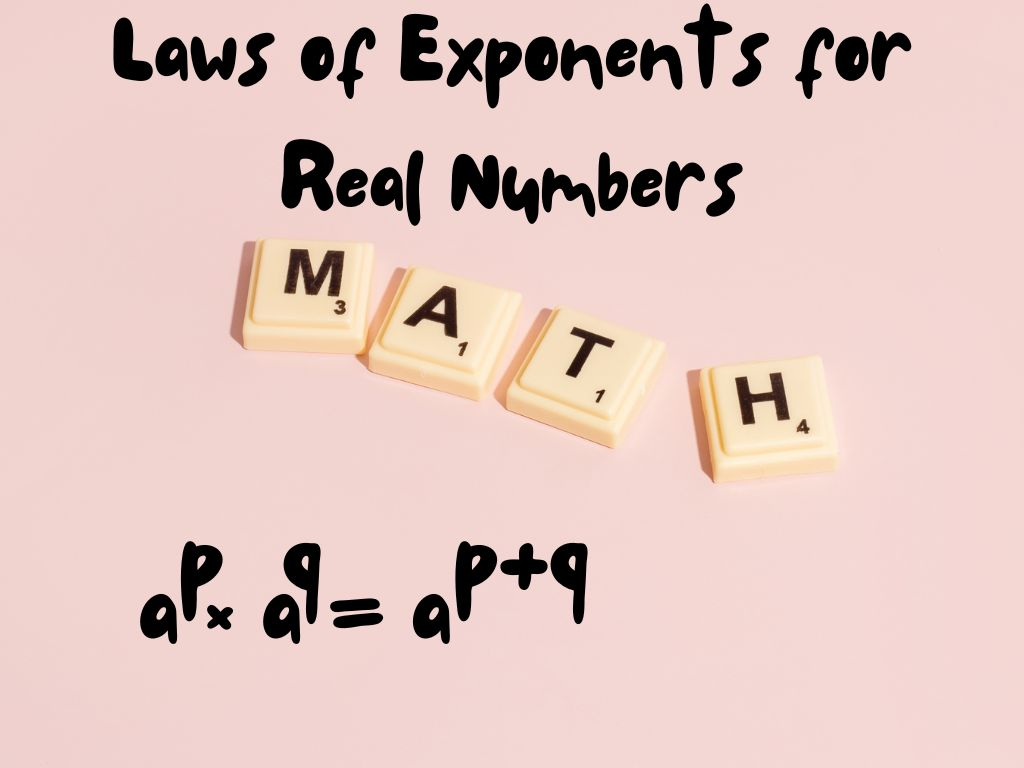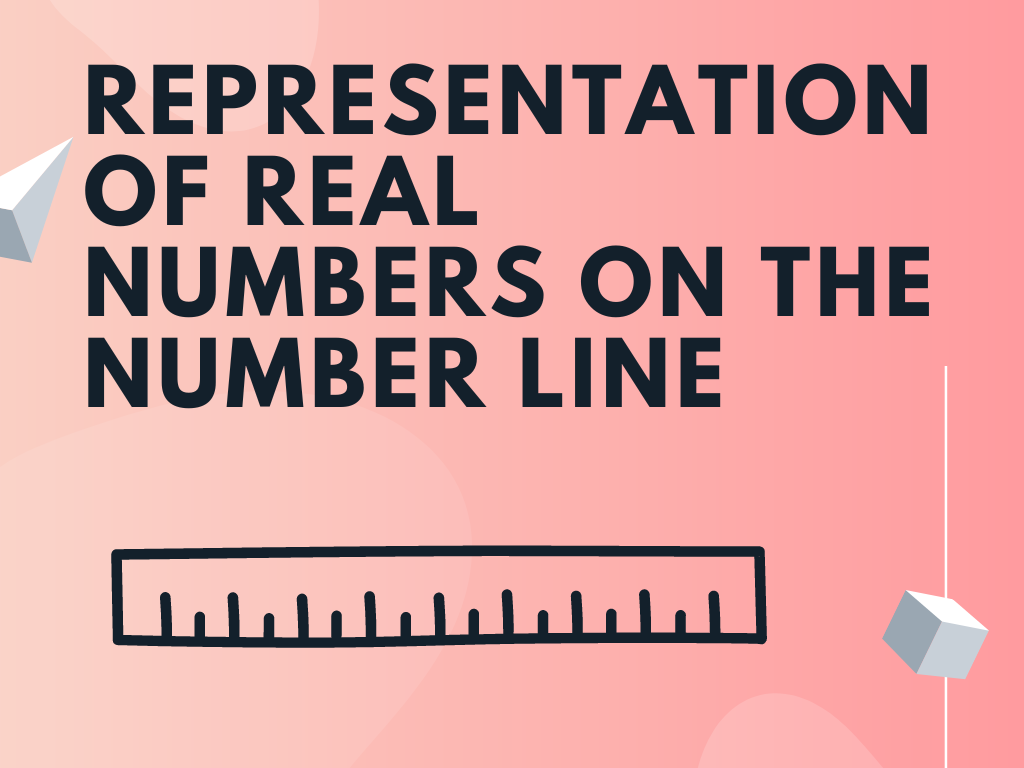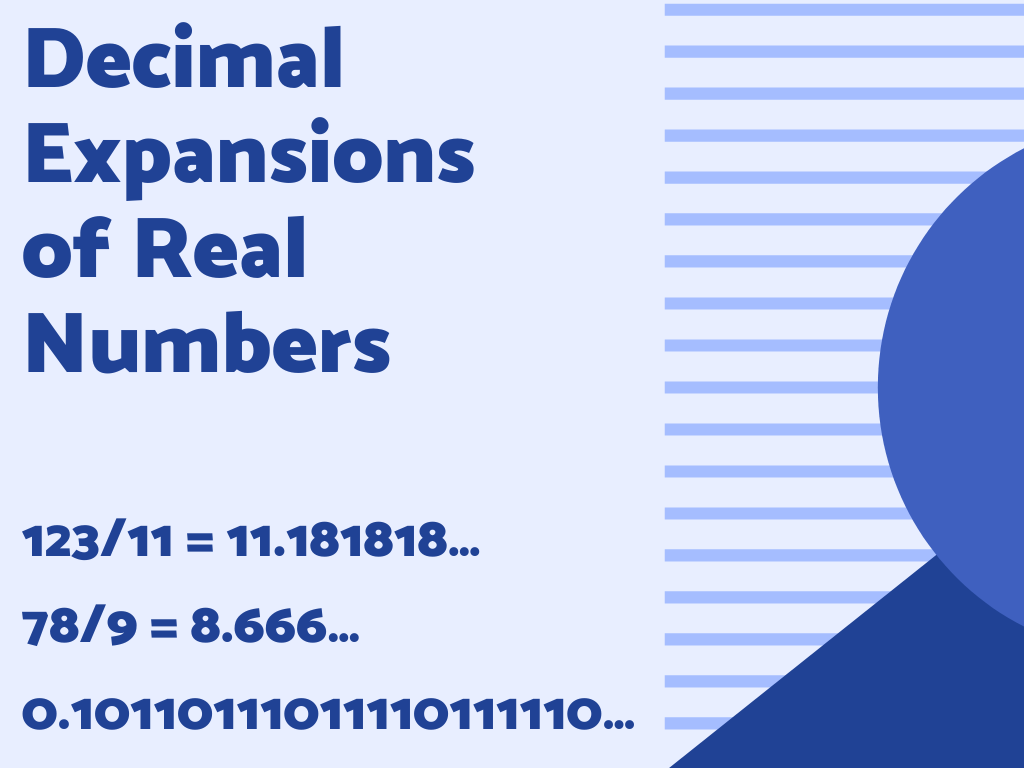# Number System Class 9th## Laws of Exponents for Real Numbers Class 9th

When we multiply a number continuously by itself till a certain number, then exponents are used to writing these numbers in a suitable and convenient form. In mathematics, we use different laws of exponents to solve large calculations. In this section, we will study different laws of exponents for real numbers. Before understanding the laws …## Operations on Real Numbers Class 9th

In this section, we shall study different mathematical operations on real numbers such as addition, subtraction, multiplication, and division. We know that rational numbers and irrational numbers are real numbers so we’ll apply operations on them. For Rational Numbers As we have learnt in previous classes, rational numbers satisfy the commutative, associative, and distributive properties …## Representation of Real Numbers on the Number Line Class 9th

As per the knowledge of the previous classes, we are familiar with the representation of different types of numbers on the number line like the representation of natural numbers, whole numbers, integers, and rational numbers. In this section, we’ll learn the representation of real numbers on the number line including irrational numbers also. We’ll represent …## Decimal Expansions of Real Numbers Class 9th

As we know that collections of all rational numbers and irrational numbers are known as real numbers. In this section, we shall study decimal expansions of real numbers means decimal expansions of rational numbers and irrational numbers. First, we start with rational numbers. Decimal Expansions of Rational Numbers Rational numbers are in the form of …